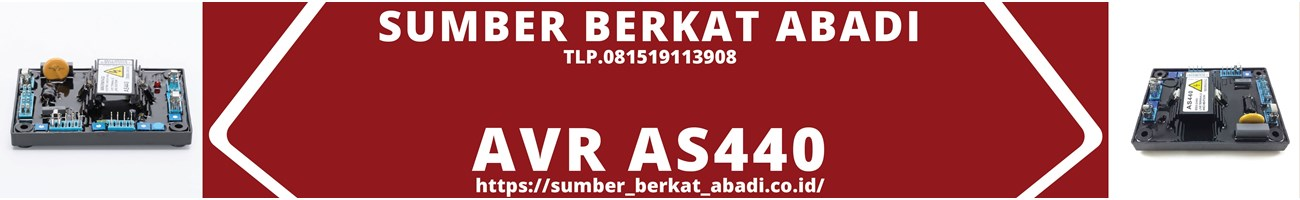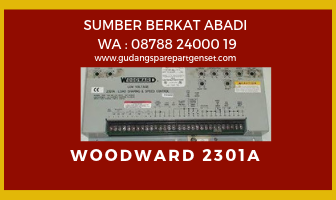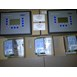Hi, Guest!
• 0LOKASI :  Jakarta Pusat

AKTIVITAS :  19 hours ago

BAGIKAN :

Bagikan :
SUMBER BERKAT ABADI GOOD QUALITY WITH COMPETITIVE PRICE. GUARANTEE YOU SATISFIED 100%

# V Belt Fan Belt 1422V300 Speed Belt

Update Terakhir
:
13 / 10 / 2020
Min. Pembelian
:
1 Set
Harga Mulai
Rp. 888.000
Sampai dengan
Rp. 999.000
Bagikan
:

## V Belt Fan Belt 1422V300 Speed Belt

Part Number = 1422V300
Length (inch) = 30.7
Length (mm) = 780
Length Type = Outside Circumference
Top Belt Width per strand (inch) = 0.88
Nominal Thickness (inch) = 0.31
RMA Oil and Heat Resistant = Yes
Core Material = Oil & Heat Resistant (chloroprene)
Tensile Cord = Polyester
Product Number = 98040493
Weight = 0.5500

## V Belt Fan Belt 1422V300 Speed Belt

Part Number = 1422V300
Length (inch) = 30.7
Length (mm) = 780
Length Type = Outside Circumference
Top Belt Width per strand (inch) = 0.88
Nominal Thickness (inch) = 0.31
RMA Oil and Heat Resistant = Yes
Core Material = Oil & Heat Resistant (chloroprene)
Tensile Cord = Polyester
Product Number = 98040493
Weight = 0.5500

## V Belt Fan Belt 1422V300 Speed Belt

Part Number = 1422V300
Length (inch) = 30.7
Length (mm) = 780
Length Type = Outside Circumference
Top Belt Width per strand (inch) = 0.88
Nominal Thickness (inch) = 0.31
RMA Oil and Heat Resistant = Yes
Core Material = Oil & Heat Resistant (chloroprene)
Tensile Cord = Polyester
Product Number = 98040493
Weight = 0.5500

## V Belt Fan Belt 1422V300 Speed Belt

Part Number = 1422V300
Length (inch) = 30.7
Length (mm) = 780
Length Type = Outside Circumference
Top Belt Width per strand (inch) = 0.88
Nominal Thickness (inch) = 0.31
RMA Oil and Heat Resistant = Yes
Core Material = Oil & Heat Resistant (chloroprene)
Tensile Cord = Polyester
Product Number = 98040493
Weight = 0.5500

## V Belt Fan Belt 1422V300 Speed Belt

Part Number = 1422V300
Length (inch) = 30.7
Length (mm) = 780
Length Type = Outside Circumference
Top Belt Width per strand (inch) = 0.88
Nominal Thickness (inch) = 0.31
RMA Oil and Heat Resistant = Yes
Core Material = Oil & Heat Resistant (chloroprene)
Tensile Cord = Polyester
Product Number = 98040493
Weight = 0.5500

belt conveyorJl. Pintu Air 3 No. 25-C Pasar Baru, Jakarta Pusat
Indonesia 10710
Jakarta Pusat
30 / 07 / 2021
Hubungi Kami

## V Belt Fan Belt 1422V300 Speed Belt

Part Number = 1422V300
Length (inch) = 30.7
Length (mm) = 780
Length Type = Outside Circumference
Top Belt Width per strand (inch) = 0.88
Nominal Thickness (inch) = 0.31
RMA Oil and Heat Resistant = Yes
Core Material = Oil & Heat Resistant (chloroprene)
Tensile Cord = Polyester
Product Number = 98040493
Weight = 0.5500

## V Belt Fan Belt 1422V300 Speed Belt

Part Number = 1422V300
Length (inch) = 30.7
Length (mm) = 780
Length Type = Outside Circumference
Top Belt Width per strand (inch) = 0.88
Nominal Thickness (inch) = 0.31
RMA Oil and Heat Resistant = Yes
Core Material = Oil & Heat Resistant (chloroprene)
Tensile Cord = Polyester
Product Number = 98040493
Weight = 0.5500

## V Belt Fan Belt 1422V300 Speed Belt

Part Number = 1422V300
Length (inch) = 30.7
Length (mm) = 780
Length Type = Outside Circumference
Top Belt Width per strand (inch) = 0.88
Nominal Thickness (inch) = 0.31
RMA Oil and Heat Resistant = Yes
Core Material = Oil & Heat Resistant (chloroprene)
Tensile Cord = Polyester
Product Number = 98040493
Weight = 0.5500

## V Belt Fan Belt 1422V300 Speed Belt

Part Number = 1422V300
Length (inch) = 30.7
Length (mm) = 780
Length Type = Outside Circumference
Top Belt Width per strand (inch) = 0.88
Nominal Thickness (inch) = 0.31
RMA Oil and Heat Resistant = Yes
Core Material = Oil & Heat Resistant (chloroprene)
Tensile Cord = Polyester
Product Number = 98040493
Weight = 0.5500

## V Belt Fan Belt 1422V300 Speed Belt

Part Number = 1422V300
Length (inch) = 30.7
Length (mm) = 780
Length Type = Outside Circumference
Top Belt Width per strand (inch) = 0.88
Nominal Thickness (inch) = 0.31
RMA Oil and Heat Resistant = Yes
Core Material = Oil & Heat Resistant (chloroprene)
Tensile Cord = Polyester
Product Number = 98040493
Weight = 0.5500

belt conveyor